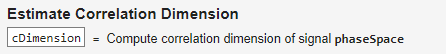# Estimate Correlation Dimension

Estimate the correlation dimension of a uniformly sampled signal in the Live Editor

Since R2019b

## Description

Correlation dimension is the measure of dimensionality of the space occupied by a set of random points. Correlation dimension is estimated as the slope of the correlation integral versus the range of radius of similarity. Use correlation dimension as a characteristic measure to distinguish between deterministic chaos and random noise, to detect potential faults.

To add the Estimate Correlation Dimension task to a live script in the MATLAB Editor:

• On the Live Editor tab, select Task > Estimate Correlation Dimension.

• In a code block in your script, type a relevant keyword, such as `correlation` or `correlation dimension`. Select `Estimate Correlation Dimension` from the suggested command completions.

## Examples

expand all

Use the Estimate Correlation Dimension task in the Live Editor to interactively estimate the correlation dimension of a uniformly sampled signal. Experiment with different values for lag, embedding dimension, similarity radius and number of points to align the linear fit line with the original data plot. The task automatically generates code reflecting your selections.

For this example, consider 'corrDimData`.mat`' which contains reconstructed phase space signal `phaseSpace`.

`load('corrDimData.mat','phaseSpace')`

To estimate the correlation dimension of the signal `phaseSpace`, open the Estimate Correlation Dimension in the Live Editor. On the Live Editor tab, select Task > Estimate Correlation Dimension. In the task, select signal `phaseSpace`.Since the selected signal is a phase space signal, select `Phase` `space` from the Signal Type menu.The Estimate Correlation Dimension task creates the correlation dimension plot with default values for the similarity radius and the number of points.If your linear fit line does not align with the original data line using the default similarity radius values, try different values in the Similarity Radius Min, Similarity Radius Max and Number of Points fields until the alignment is satisfactory. For this example, use the minimum value of 0.08 and maximum value of 5 for the best alignment. The default value of 10 points provides good alignment for the signal `phaseSpace`.You can toggle displaying the output of the correlation dimension value in the Live Editor output using the Output Display option.The task generates code in your live script. The generated code reflects the parameters and options you specify. To see the generated code, clickbeneath the Display Results selection. The task expands to display the generated code.By default, the generated code uses `corrDim` as the name of the output variable. To specify a different output variable name, enter a new name in the summary line at the top of the task. For instance, change the name to `cDimension`.The task updates the generated code to reflect the new variable name, and the new variable `cDimension` appears in the MATLAB workspace. The value of correlation dimension is directly proportional to the level of chaos in the system, that is, a higher value of `cDimension` represents a high level of chaotic complexity in the system.

## Parameters

expand all

Select Signal

Select a uniformly sampled time-domain signal in array or timetable format from the MATLAB workspace. If the signal has multiple columns, the Estimate Correlation Dimension task computes the correlation dimension by treating it as a multivariate signal. If the signal is a row vector, then the Estimate Correlation Dimension task treats it as a univariate signal.

Specify the type of the selected signal as either '`Time Domain`' or '`Phase space`'. If you specify the signal type as:

• '`Time Domain`', then also specify the embedding dimension and time lag for your signal.

• '`Phase space`', then the Estimate Correlation Dimension task automatically infers the embedding dimension and time lag using the phase space information.

Specify Correlation Dimension Parameters

Specify the number of dimensions of phase space vectors as a scalar or vector from the MATLAB workspace. When you specify the embedding dimension as a scalar, then the Estimate Correlation Dimension task uses the same embedding dimension value to estimate the value of correlation dimension for all the columns of the uniformly sampled signal.

The `Embedding Dimension` drop down is active only when you specify the signal type as '`Time Domain`'. For phase space signals, the Estimate Correlation Dimension task automatically computes the embedding dimension from the phase space data.

If you do not know the value of embedding dimension for your signal, then you can compute it using the Reconstruct Phase Space task.

Specify time lag between successive phase vectors as a scalar or vector from the MATLAB workspace. When you specify the time lag as a scalar, then the Estimate Correlation Dimension task uses the same time delay value to estimate the value of correlation dimension for all the columns of the uniformly sampled signal. If you specify the embedding dimension as a vector, then specify the time lag also as a vector of the same length.

The `Time Lag` drop down is active only when you specify the signal type as '`Time Domain`'. For phase space signals, the Estimate Correlation Dimension task automatically computes the time lag from the phase space data.

If you do not know the value of time lag for your signal, then you can compute it using the Reconstruct Phase Space task.

Specify the minimum radius of similarity to be used to compute the number of with-in range points for correlation dimension estimation. Try different values such that the linear fit line aligns with the original data line in the plot.

Specify the maximum radius of similarity to be used to compute the number of with-in range points for correlation dimension estimation. Try different values such that the linear fit line aligns with the original data line in the plot.

Specify the number of points between the maximum and minimum radius of similarity. Choose an appropriate number of points based on the resolution required to compute the correlation dimension.

Visualize Results

Toggle to display the value of correlation dimension in the Live Editor output.

## Version History

Introduced in R2019b